### Iterative Methods: Jacobi Method

### Introduction

In the previous section, we introduced methods that produced an exact solution for the determined linear system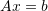. These methods relied on exactly solving the set of equations at hand. There are other “numerical techniques” that involve iterative methods that are similar to the iterative methods shown in the root finding methods section. Whenis relatively large, and when the matrix is banded, then these methods might become more efficient than the traditional methods above.

### The Jacobi Method

The Jacobi method is named after Carl Gustav Jacob Jacobi. The method is akin to the fixed-point iteration method in single root finding described before. First notice that a linear systemof sizecan be written as: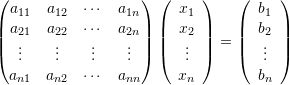The left hand side can be decomposed as follows: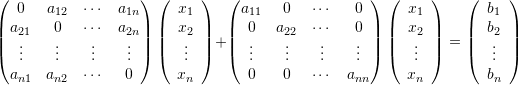Effectively, we have separated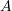into two additive matrices: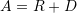where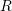has zero entries in the diagonal components and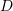is a diagonal matrix. If we start with nonzero diagonal components for, thenis a diagonal matrix with nonzero entries in the diagonal and can easily be inverted and its inverse is: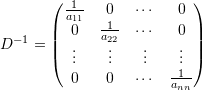Therefore: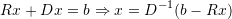This form is similar to the fixed-point iteration method. By assuming initial guesses for the components of the vectorand substituting in the right hand side, then a new estimate for the components ofcan be computed. In addition to having non-zero diagonal components for, there are other requirements for the matrix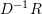for this method to converge to a proper solution which are beyond the scope of these notes. One fact that is useful is that this method will converge if the diagonal components ofare large compared to the rest of the matrix components. The criteria for stopping this algorithm will be based on the size or the norm of the difference between the vectorin each iteration. For this, we can use the Euclidean norm. So, if the components of the vectorafter iterationare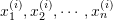, and if after iteration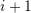the components are: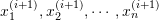, then, the stopping criterion would be: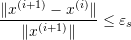where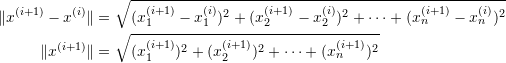Note that any other norm function can work as well.

#### Example

Consider the systemdefined as: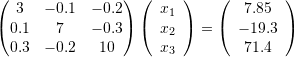The Jacobi method with a stopping criterion of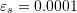will be used. First the system is rearranged to the form: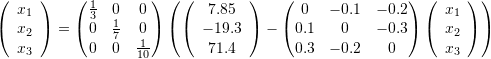Then, the initial guesses for the components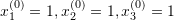are used to calculate the new estimates: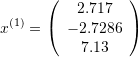The relative approximate error in this case is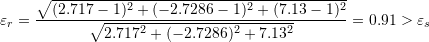The next iteration: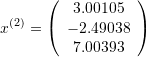The relative approximate error in this case is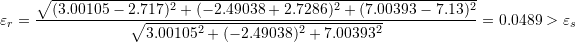The third iteration: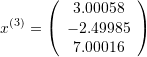The relative approximate error in this case is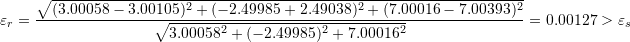The fourth iteration: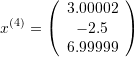The relative approximate error in this case is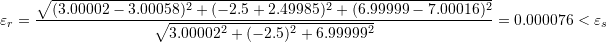Therefore convergence has been achieved. The exact solution is in fact: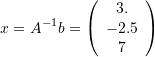### Programming the Method

We will use the built-in Norm function for the stopping criteria. In the following code, the procedure “J” takes the matrix, the vector, and the guessto return a new guess for the vector. The maximum number of iterations is 100 and the stopping criteria are either the maximum number of iterations is reached or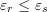:

View Mathematica Code
J[A_, b_, x_] := (
n = Length[A];
InvD = Table[0, {i, 1, n}, {j, 1, n}];
R = A;
Do[InvD[[i, i]] = 1/A[[i, i]]; R[[i, i]] = 0, {i, 1, n}];
newx = InvD.(b - R.x)
)

A = {{3, -0.1, -0.2}, {0.1, 7, -0.3}, {0.3, -0.2, 10}}
b = {7.85, -19.3, 71.4}
x = {{1, 1, 1.}};
MaxIter = 100;
ErrorTable = {1};
eps = 0.001;
i = 2;

While[And[i <= MaxIter, Abs[ErrorTable[[i - 1]]] > eps],
xi = J[A, b, x[[i - 1]]]; x = Append[x, xi];
ei = Norm[x[[i]] - x[[i - 1]]]/Norm[x[[i]]];
ErrorTable = Append[ErrorTable, ei]; i++]
x // MatrixForm
ErrorTable // MatrixForm

View Python Code
import numpy as np
def J(A, b, x):
A = np.array(A)
n = len(A)
InvD = np.zeros([n,n])
R = A
for i in range(n):
InvD[i, i] = 1/A[i, i]
R[i, i] = 0
return np.matmul(InvD,(b - np.matmul(R,x)))

A = [[3, -0.1, -0.2], [0.1, 7, -0.3], [0.3, -0.2, 10]]
b = [7.85, -19.3, 71.4]
x = [[1, 1, 1.]]
MaxIter = 100
ErrorTable = 
eps = 0.001
i = 1

while i <= MaxIter and abs(ErrorTable[i - 1]) > eps:
xi = J(A, b, x[i - 1])
x.append(xi)
ei = np.linalg.norm(x[i] - x[i - 1])/np.linalg.norm(x[i])
ErrorTable.append(ei)
i+=1
print("x:",np.array(x))
print("ErrorTable:",np.vstack(ErrorTable))


### Lecture Video

The following video covers the Jacobi method.